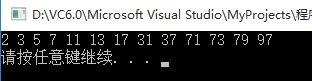# 程序基本算法习题解析 求出100之内的所有可逆素数

2023-09-25 7 0

``````// Chapter5_4.cpp : Defines the entry point for the application.
// 求出100之内的所有可逆素数
// 可逆素数是指一个素数将其各位数字的顺序倒过来构成的反序数也是素数#include "stdafx.h"
#include<iostream>
#include<math.h>
using namespace std;
const int N = 99;
//判断是否为素数，是素数返回1，不是素数返回0
int isPrime(int x)
{//因为后面的for循环里面有sq，i的初始值又是从2开始，因此将2,3单独进行判断if(x==2 || x==3)  return 1;else{int sq = sqrt(x);for(int i=2;i<=sq;i++){if(x%i == 0)return 0;}return 1;}
}
//判断是否为可逆素数,是可逆素数返回1，不是可逆素数返回0
//要求输入为素数
int isReverPrime(int x)
{if((x/10) == 0) //如果是一位数return 1; //是可逆素数else{int shi,ge,reverse;shi = x/10;  //提取十位数ge = x - shi*10;  //提取各位数reverse = ge*10+shi;//判断逆数是否为素数if(isPrime(reverse))return 1;elsereturn 0;}
}
//主函数
int main()
{//声明一个二维数组，第一行放值，第二行放素数判断情况（是素数赋为1，不是素数赋为0）int a[N+1],i; for(i=0;i<N+1;i++)a[i] = i;for(i=2;i<N+1;i++){//如果是素数if(isPrime(a[i])){a[i] = 1; //对应的下一行位置置1if(a[i])if(isReverPrime(a[i])) //如果是可逆素数cout << a[i] << ' '; //输出可逆素数}}cout << endl;system("pause");return 0;
}``````